# Override Equals Method in C#

## Override Equals Method in C# with Examples

In this article, I am going to discuss Why we need to Override the Equals Method in C# with Examples. Please read our previous article before proceeding to this article where we discussed Why and How to Override the ToString() Method in C#. As part of this article, we are going to discuss the following pointers.

1. Understanding the Equals Method of Object class in C#?
2. Understanding the difference between the “==” Operator and the Equals() Method in C#?
3. Why do we need to override the Equals() Method in C#?
4. How we can override the Equals Method in C# with Examples?
##### What is Equals Method in C#?

The Equals is a virtual method defined in the Object class and this method is available to all the .NET Types as Object is the superclass of all .NET Types.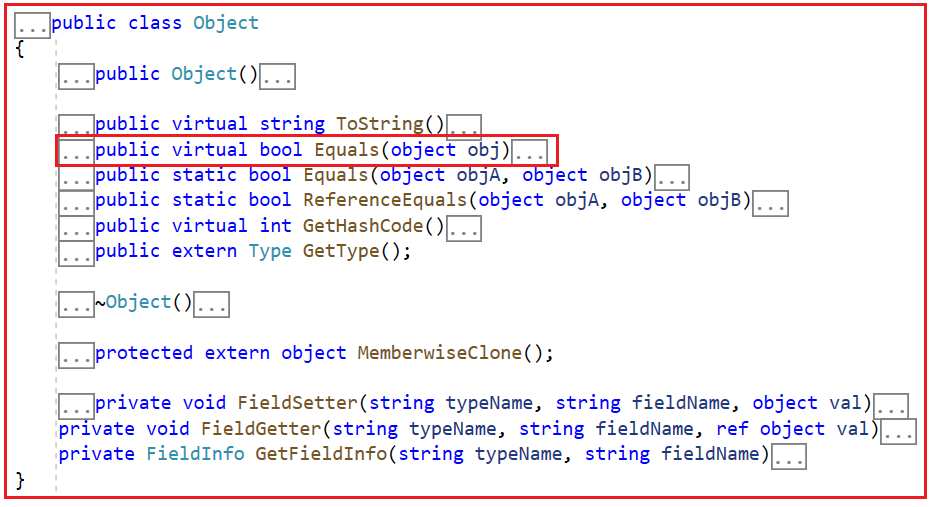As Equals is a virtual method, we can also override this method under our classes. The following is the signature of this method.

1. public virtual bool Equals(object obj): This method is used to determine whether the specified object is equal to the current object. Here, the parameter obj specifies the object to compare with the current object. It returns true if the specified object is equal to the current object; otherwise, false.

Before understanding how to override and when to override, let us first understand what is the difference between the == Operator and Equals method in C#.

##### Difference Between the “==” Operator and the Equals() Method in C#:

As we already discussed every type in .NET, directly or indirectly inherits from the Object class. So, the Equals() virtual method, which has a default implementation within the Object class is also available in every .NET (Primitive as well as Reference) type via inheritance.

In the following example, the variables Number1 and Number2 are integers. So, both the == and Equals() method returns true, since Number1 and Number2, both variables have the same value i.e. 10. Integers are value types and they hold the value directly, and hence we get the result true in this case.

```using System;
namespace UnderstandingEqualsMethod
{
public class Program
{
public static void Main()
{
int Number1 = 10;
int Number2 = 10;
Console.WriteLine(\$"Number1 == Number2: {Number1 == Number2}");
Console.WriteLine(\$"Number1.Equals(Number2): {Number1.Equals(Number2)}");
}
}
}
```
###### Output: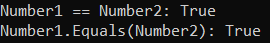The following diagram shows the memory architecture of the above program. As integer is a value type, so they are holding the value directly, and, in this case, both the == operator and Equals method checks the values and find it to be TRUE.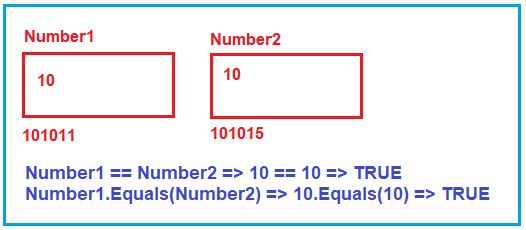##### Equals method and == Operator with Enum Type in C#:

In the following example, we compare 2 enums, and both the == operator and Equals() method return true since both direction1 and direction2 enums have the same underlying integer value i.e. 1. Ang again, Enums are value type and they hold the value instead of the reference address.

```using System;
namespace UnderstandingEqualsMethod
{
public class Program
{
public static void Main()
{
Direction direction1 = Direction.East;
Direction direction2 = Direction.East;
Console.WriteLine(direction1 == direction2);
Console.WriteLine(direction1.Equals(direction2));
}
}
public enum Direction
{
East = 1,
West = 2,
North = 3,
South = 4
}
}
```
###### Output: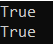##### Equals method and == Operator with Reference Type in C#:

If the type is a reference type, then by default both the == operator and Equals method check for reference equality whereas we can change this default behavior of the Equals() method by overriding it to check for value equality. If this is not clear at the moment, don’t worry let us understand this with an example,

In the following example, C1 and C2 are 2 different object reference variables of the Customer class. But both are pointing to the same object. The most important point that you need to keep in mind is reference variables are different from objects. Reference variables are created on the stack memory and they point to the actual objects which are stored in the heap memory.

Since, C1 and C2 both refer to the same object, the reference equality, and the value equality is true. Value equality means that two objects contain the same values. In this example, the actual object is only one, so obviously, the values are also equal. If two objects have reference equality, then they also have value equality, but value equality does not guarantee reference equality.

```using System;
namespace UnderstandingEqualsMethod
{
public class Program
{
public static void Main()
{
Customer C1 = new Customer();
C1.FirstName = "Pranaya";
C1.LastName = "Rout";

Customer C2 = C1;

Console.WriteLine(\$"C1 == C2: {C1 == C2}");
Console.WriteLine(\$"C1.Equals(C2): {C1.Equals(C2)}");

}
}
public class Customer
{
public string FirstName { get; set; }
public string LastName { get; set; }
}
}
```
###### Output: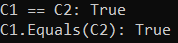The following diagram shows the memory architecture of the above program. In this case, the actual Customer object is created inside the Heap Memory and in Stack memory, two customer reference variables are created and both of them point to the same customer object base address. As both C1 and C2 hold the same customer object reference and hence, both == operator and Equals method check the references and find it to be TRUE.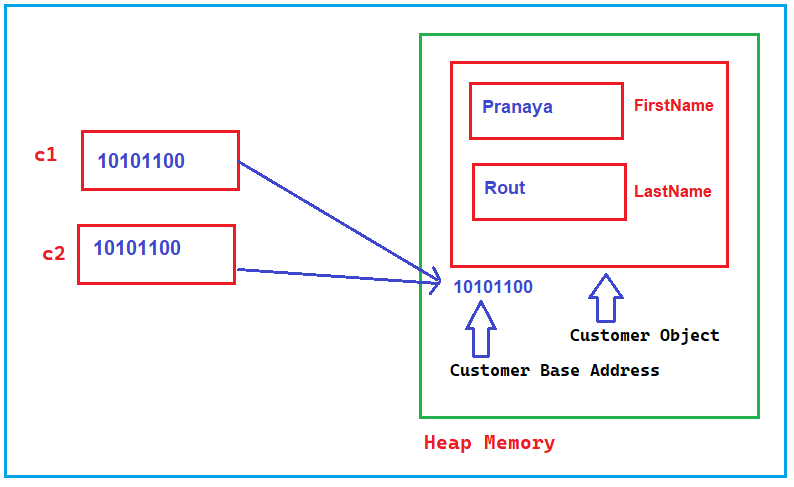In the following example, the == operator returns False. This makes sense because C1 and C2 are referring to different objects. However, the Equals() method also returns false, in spite of the values across C1 and C2 being the same and this is because by default Equals method checks the reference equality.

```using System;
namespace UnderstandingEqualsMethod
{
public class Program
{
public static void Main()
{
Customer C1 = new Customer();
C1.FirstName = "Pranaya";
C1.LastName = "Rout";

Customer C2 = new Customer();
C2.FirstName = "Pranaya";
C2.LastName = "Rout";

Console.WriteLine(\$"C1 == C2: {C1 == C2}");
Console.WriteLine(\$"C1.Equals(C2): {C1.Equals(C2)}");

}
}
public class Customer
{
public string FirstName { get; set; }
public string LastName { get; set; }
}
}
```
###### Output: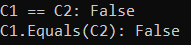The following diagram shows the memory architecture of the above program. In this case, we have created two Customer objects inside the Heap Memory and in Stack memory, we have two customer reference variables and both are pointing to different customer objects. As both C1 and C2 hold different customer object references and hence, both == operator and Equals methods check the references and find it to be FALSE.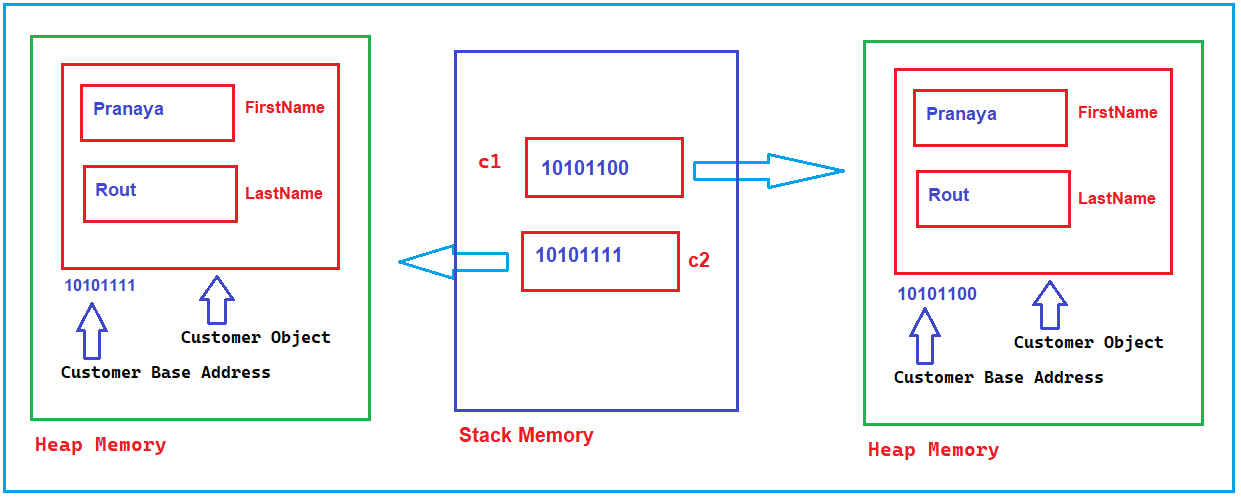Now, if you want the Equals method to check the values stored inside the object instead of the reference address, then we need to override the Equals method inside the Customer class and if the value is equal then we need to return TRUE.

##### Overriding the Equals Method of the Object Class in C#:

In the following example, we override the Equals() method of the Object class inside the Customer class. When overriding the Equals() method, make sure the passed object is not null and can be cast to the type you are comparing. When overriding Equals(), you also need to override GetHashCode(), otherwise you will get a compiler warning.

```using System;
namespace UnderstandingEqualsMethod
{
public class Program
{
public static void Main()
{
Customer C1 = new Customer();
C1.FirstName = "Pranaya";
C1.LastName = "Rout";

Customer C2 = new Customer();
C2.FirstName = "Pranaya";
C2.LastName = "Rout";

Console.WriteLine(\$"C1 == C2: {C1 == C2}");
Console.WriteLine(\$"C1.Equals(C2): {C1.Equals(C2)}");

}
}
public class Customer
{
public string FirstName { get; set; }
public string LastName { get; set; }

public override bool Equals(object obj)
{
// If the passed object is null, return False
if (obj == null)
{
return false;
}
// If the passed object is not Customer Type, return False
if (!(obj is Customer))
{
return false;
}
return (this.FirstName == ((Customer)obj).FirstName)
&& (this.LastName == ((Customer)obj).LastName);
}
}
}
```
###### Output: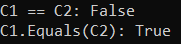Now, the Equals method will not check the reference address instead it will check the First Name and Last Name of both the objects and if it is found to be the same, then it will return TRUE else it will return FALSE. Further, if you look at the Customer class, then it is showing one warning as shown in the below image.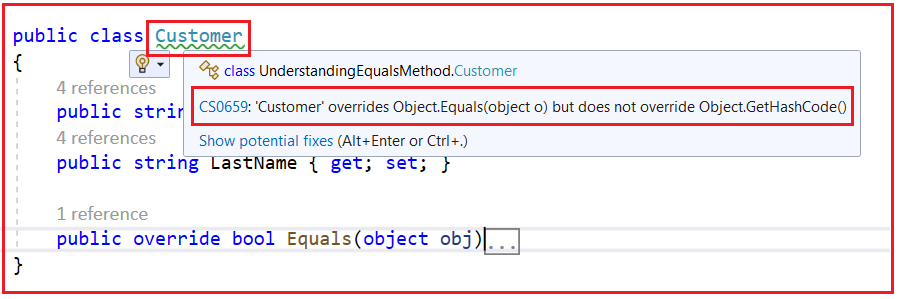Here, the compiler complains that you have to override the Equals method in the Customer class, but you have not overridden the GetHashCode method. So, it is not mandatory to override the GetHashCode method, but it is recommended to override the GetHashCode method if you are overriding the Equals method in C#. Even using the GetHashCode method, we can also check whether two objects are equal or not which is shown in the below example.

```using System;
namespace UnderstandingObjectClassMethods
{
public class Program
{
public static void Main()
{
Customer C1 = new Customer();
C1.FirstName = "Pranaya";
C1.LastName = "Rout";

Customer C2 = new Customer();
C2.FirstName = "Pranaya";
C2.LastName = "Rout";

var hashcode1 = C1.GetHashCode();
var hashcode2 = C2.GetHashCode();

Console.WriteLine(\$"C1.GetHashCode == C2.GetHashCode:{hashcode1 == hashcode2}");
Console.WriteLine(\$"C1 == C2:{C1 == C2}");
Console.WriteLine(\$"C1.Equals(C2):{C1.Equals(C2)}");

}
}
public class Customer
{
public string FirstName { get; set; }
public string LastName { get; set; }

public override bool Equals(object obj)
{
// If the passed object is null
if (obj == null)
{
return false;
}
if (!(obj is Customer))
{
return false;
}
return (this.FirstName == ((Customer)obj).FirstName)
&& (this.LastName == ((Customer)obj).LastName);
}

//Overriding the GetHashCode method
//GetHashCode method generates hashcode for the current object
public override int GetHashCode()
{
//Performing BIT wise OR Operation on the generated hashcode values
//If the corresponding bits are different, it gives 1.
//If the corresponding bits are the same, it gives 0.
return FirstName.GetHashCode() ^ LastName.GetHashCode();
}
}
}
```
###### Output: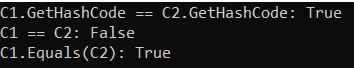In the next article, I am going to discuss the difference between the Convert.ToString and ToString() method in C#.  In this article, I try to explain why we should override the Equals Method in C# with Examples. I hope you understood why and how to override the Equals method in C#.

### 10 thoughts on “Override Equals Method in C#”

1.Mohamed Hassan

Sir, I did not understand this part

public override int GetHashCode()
{
return FirstName.GetHashCode() ^ LastName.GetHashCode();
}
Can you explain more about why we are using GetHashCode() and what is the ^ symbol is used for?

1.Süleyman Güvenç

I thing compiler is warning us that we may need to override GetHashCode, Sometimes we may check equality with GetHashCode metod. In that sample Console.WriteLine(C1.GetHashCode() == C2.GetHashCode()); exposes true result. İf we dont add this overriding, this checking would return false.

2.Süleyman Güvenç

I thing compiler is warning us that we may need to override GetHashCode, Sometimes we may check equality with GetHashCode metod. In that sample Console.WriteLine(C1.GetHashCode() == C2.GetHashCode()); exposes true result. İf we dont add this overriding, this checking would return false.

3.using System;

public class Program
{
public static void Main()
{
Customer C1 = new Customer();
C1.FirstName = “Pranaya”;
C1.LastName = “Rout”;

Customer C2 = C1;

Console.WriteLine(C1 == C2);
Console.WriteLine(C1.Equals(C2));
}
}
public class Customer
{
public string FirstName { get; set; }
public string LastName { get; set; }
}
I think both should return true.

1.Gagandeep Singh

Yes you are right

2.dont think actually both return true

1.They are both referencing the same object so it should return true

4.public class Program
{
public static void Main()
{
Customer C1 = new Customer();
C1.FirstName = “Pranaya”;
C1.LastName = “Rout”;

Customer C2 = new Customer();
C2.FirstName = “Pranaya”;
C2.LastName = “Rout”;

Console.WriteLine(C1 == C2);
Console.WriteLine(C1.Equals(C2));
}
}
How .Equals() return false? Because both have same values and .Equals() goes for value equality.

1.Both C1 and C2 are instances of different object pointing to different memory locations that’s why Equal() method is returning FALSE value even though it checks for value equality.

5.